Strange things happen to an expression containing a variable on which assumptions have been made, when those assumptions are changed or a value is assigned to the variable. For example:

> assume(x > 0);

> a:= x+1:

> a-x;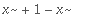This effect is due to the fact that when an assumption is made about a variable, the name of that variable is changed. If the "Trailing Tildes" option for assumed variables is being used (which is the default), the new name is printed with a ~ . The name change occurs even without this option (the option has only a cosmetic effect, changing how the name is printed) . This is an illustration of the fact that two names that look the same to us can be different to Maple.

> assume(x,integer);

> x;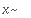Originally x, renamed x~:
is assumed to be: integer

The old name is assigned the new name as its value. Thus when you enter and evaluate x , you obtain the new name.

> 'x'-x;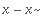Here we delayed evaluation of the first x by putting it in forward quotes. The second x was evaluated normally. The result is the difference of two distinct variables, instead of 0. If evaluation is allowed to occur (e.g. by recalling the last result), we do get 0.

> ";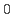The new name can't be entered directly from the keyboard: it is not the same as what you get by entering the string `x~`.

> `x~`-x;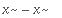Now if a new assumption is made about x using assume or additionally , we get another new name x~ that is different from the previous one. The original x is assigned this as a value, so when any expression that contains the original x is evaluated the new x~ will be used. But the previous x~ is left unchanged, so that evaluation of an expression that contains it will not use the new x~ . Similarly, if you assign x a value you are not affecting expressions that contain x~ .

A cure for these problems is to assign the new x~ or the new value to the old x~ as well as to x . It can be done as follows. Note that we must save the name of the old x~ in a variable before changing the assumption. We then use assign rather than := because we want to make an assignment to the value of oldx (which is the name of the old x~ ) rather than to oldx itself.

> assume(x > 0);
a:= x+1:

> oldx:= x:
assign(oldx,x):

> a-x;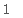Similarly, this technique will work when x is being assigned a value. But the simplest thing to do in that case is to assign the value with assign rather than := .

> assign(x,2);

> a;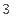Maple Advisor Database, R. Israel 1997